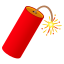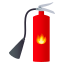# Construct circuit that implements truth table

#### evinda

##### Well-known member
MHB Site Helper
Hello!!!I want to construct a circuit that implements the following truth table:

\begin{equation*}
\begin{array}{c|c|c|c|c}
P(Input) & Q(Input) & K(Output) & A(Output) \\
\hline
1 & 1 & 1 & 0 \\
1 & 0 & 0 & 1 \\
0 & 1 & 0 & 1 \\
0 & 0 & 0 & 0
\end{array}
\end{equation*}

In addition, I want to use the above circuit to construct a full adder, i.e. a circuit that adds three 1-digit binary numbers.

Could you help me to construct the wanted circuit that implements the truth table?•Klaas van Aarsen

#### Klaas van Aarsen

##### MHB Seeker
Staff member
Hey evinda !!

Isn't output K the result of an AND port?
And output A the result of a XOR port?
Can we construct a circuit from those?To add three 1-digit binary numbers, we need a truth table with inputs P, Q, R, and outputs K, A, don't we?•evinda, topsquark and MarkFL

#### evinda

##### Well-known member
MHB Site Helper
Hey evinda !!

Isn't output K the result of an AND port?
And output A the result of a XOR port?
Can we construct a circuit from those?The XOR port gives $1$ when the input of true inputs is odd and $0$ otherwise, right?Can you help me construct the desired circuit? Do we create circles that represent the AND and XOR port and create arrows representing each possible input and ending at the output of the port? Or do we do something else?#### Klaas van Aarsen

##### MHB Seeker
Staff member
The XOR port gives $1$ when the input of true inputs is odd and $0$ otherwise, right?
Yep.Can you help me construct the desired circuit? Do we create circles that represent the AND and XOR port and create arrows representing each possible input and ending at the output of the port? Or do we do something else?
The are usually drawn as shown in the wiki article about Logic Gates.•evinda

#### evinda

##### Well-known member
MHB Site Helper
The are usually drawn as shown in the wiki article about Logic Gates.So you mean we construct the circuits as follows?If so, does this mean that the we distinguish the circuits, i.e. what they represent, by taking into consideration the form ?#### Klaas van Aarsen

##### MHB Seeker
Staff member
So you mean we construct the circuits as follows?
Yep.If so, does this mean that the we distinguish the circuits, i.e. what they represent, by taking into consideration the form ?
What do you mean?Distinguish which circuits?
Consider which form?

•evinda

#### evinda

##### Well-known member
MHB Site Helper
What do you mean?Distinguish which circuits?
Consider which form?
I meant the boxes that take the input. Do we look at the form of the boxes to see which logical operation we have?For example, at the above circuits the only difference is the sumbol of the output and the form of the box... Isn't it?#### Klaas van Aarsen

##### MHB Seeker
Staff member
I meant the boxes that take the input. Do we look at the form of the boxes to see which logical operation we have? For example, at the above circuits the only difference is the sumbol of the output and the form of the box... Isn't it?
Yes, the shapes of the boxes identify the operations.
And the letters identify the inputs and the outputs.•evinda

#### evinda

##### Well-known member
MHB Site Helper
To add three 1-digit binary numbers, we need a truth table with inputs P, Q, R, and outputs K, A, don't we?We need to get the outputs K,A in order to use thae above circuit?Also do we put the two circuits into one box so that it is joined-up?#### Klaas van Aarsen

##### MHB Seeker
Staff member
We need to get the outputs K,A in order to use thae above circuit?

Also do we put the two circuits into one box so that it is joined-up?
Yes, put the two logic gates into 1 box, which will have inputs P and Q, and outputs K and A.
Afterwards I guess we'll have to reuse that box multiple times to achieve adding 3 binary bits.•evinda

#### evinda

##### Well-known member
MHB Site Helper
Yes, put the two logic gates into 1 box, which will have inputs P and Q, and outputs K and A.
So we just draw a box including the both circuits above? Then the circuits would be independent one an other.... Or do we connect them somehow?#### Klaas van Aarsen

##### MHB Seeker
Staff member
So we just draw a box including the both circuits above? Then the circuits would be independent one an other.... Or do we connect them somehow?
The surrounding box should have only 2 inputs.
Inside the box those inputs should be split and go to both of the logic gates.•evinda

#### evinda

##### Well-known member
MHB Site Helper
The surrounding box should have only 2 inputs.
Inside the box those inputs should be split and go to both of the logic gates.You mean that the circuit has the following form?Or do I have to change something?#### Klaas van Aarsen

##### MHB Seeker
Staff member
P and Q should not be connected to each other should they?Instead each of them should go separately to each logic gate.•evinda

#### evinda

##### Well-known member
MHB Site Helper
P and Q should not be connected to each other should they?Instead each of them should go separately to each logic gate.I have tried several times to draw such a circuit, but can it happen that the arrows of P and Q do not meet?#### Klaas van Aarsen

##### MHB Seeker
Staff member
\begin{tikzpicture}

\usetikzlibrary{shapes.gates.logic.US}
\tikzstyle{branch}=[fill,shape=circle,minimum size=3pt,inner sep=0pt]

\node (P) at (-1,2) {P};
\node (Q) at (-1,1) {Q};
\coordinate (Pbranch) at (0.5,2) {};
\coordinate (Qbranch) at (1,1) {};

\node[and gate US, draw, logic gate inputs=nn] at (2,2) (And1) {};
\node[xor gate US, draw, logic gate inputs=nn] at (2,1) (Xor1) {};

\draw (P) -- (Pbranch) node[branch] {};
\draw (Q) -- (Qbranch) node[branch] {};
\draw (Pbranch) |- (And1.input 1);
\draw (Pbranch) |- (Xor1.input 1);
\draw (Qbranch) |- (And1.input 2);
\draw (Qbranch) |- (Xor1.input 2);
\draw (And1.output) -- +(2,0) node[ right ] { K };
\draw (Xor1.output) -- +(2,0) node[ right ] { A };

\draw[thick] (0,0) rectangle (3,3);

\end{tikzpicture}•evinda

#### evinda

##### Well-known member
MHB Site Helper
\begin{tikzpicture}

\usetikzlibrary{shapes.gates.logic.US}
\tikzstyle{branch}=[fill,shape=circle,minimum size=3pt,inner sep=0pt]

\node (P) at (-1,2) {P};
\node (Q) at (-1,1) {Q};
\coordinate (Pbranch) at (0.5,2) {};
\coordinate (Qbranch) at (1,1) {};

\node[and gate US, draw, logic gate inputs=nn] at (2,2) (And1) {};
\node[xor gate US, draw, logic gate inputs=nn] at (2,1) (Xor1) {};

\draw (P) -- (Pbranch) node[branch] {};
\draw (Q) -- (Qbranch) node[branch] {};
\draw (Pbranch) |- (And1.input 1);
\draw (Pbranch) |- (Xor1.input 1);
\draw (Qbranch) |- (And1.input 2);
\draw (Qbranch) |- (Xor1.input 2);
\draw (And1.output) -- +(2,0) node[ right ] { K };
\draw (Xor1.output) -- +(2,0) node[ right ] { A };

\draw[thick] (0,0) rectangle (3,3);

\end{tikzpicture}Seems reasonable...What do the dots represent?#### Klaas van Aarsen

##### MHB Seeker
Staff member
Seems reasonable... What do the dots represent?
A dot shows that the lines are connected. It's like they are soldered together.
Without a dot the lines cross without connection.•evinda

#### evinda

##### Well-known member
MHB Site Helper
A dot shows that the lines are connected. It's like they are soldered together.
Without a dot the lines cross without connection.What would it be if the dots would be missing at the specific case? So that I understand it better...#### Klaas van Aarsen

##### MHB Seeker
Staff member
What would it be if the dots would be missing at the specific case? So that I understand it better...
The same thing since in this particular case since the lines are not crossing where the dots are.
So they are connected anyway. In this case we could leave out the 2 dots. They merely clarify that the line crossings are not connected.Last edited:
•evinda

#### evinda

##### Well-known member
MHB Site Helper
The same thing since in this particular case since the lines are not crossing where the dots are.
So they are connected anyway. In this case we could leave out the 2 dots. They merely clarify that the line crossings are not connected.And what would happen if we would place dots at the point where the arrows of P and Q intersect?I am confused right now....#### Klaas van Aarsen

##### MHB Seeker
Staff member
And what would happen if we would place dots at the point where the arrows of P and Q intersect? I am confused right now....
Then those lines would be connected and current can flow directly between the inputs P and Q.
It means we have a short circuit and if there are any fuses around they will blow up.
Or otherwise a fire will be started.
It is bad.•evinda

#### evinda

##### Well-known member
MHB Site Helper
Then those lines would be connected and current can flow directly between the inputs P and Q.
It means we have a short circuit and if there are any fuses around they will blow up.
Or otherwise a fire will be started.
It is bad.So in this case we just draw them in order to emphasize the different directions that the inputs go, they do not represent anything about the intersection of the different inputs, right?Also, according to what you said , in any circuit of the logical expressions, we don't have dots at the intersection points. Or am I wrong?#### Klaas van Aarsen

##### MHB Seeker
Staff member
So in this case we just draw them in order to emphasize the different directions that the inputs go.
Yes...They do not represent anything about the intersection of the different inputs, right?
Different inputs should never intersect. It would mean we have a short circuit.Also, according to what you said , in any circuit of the logical expressions, we don't have dots at the intersection points. Or am I wrong?
We can have dots... I guess it may be a matter of preference...
The important thing is that there is no ambiguity...Here's a different way to eliminate any ambiguity.
\begin{tikzpicture}
\usetikzlibrary{shapes.gates.logic.US}

\node[ and gate US, draw, logic gate inputs=nn ] at (2,2) (And1) {};
\node[ xor gate US, draw, logic gate inputs=nn ] at (2,1) (Xor1) {};

\draw (And1.input 1) -- +(-2.5,0) node[ left ] {P} +(-1.5,0) |- (Xor1.input 1);
\draw (Xor1.input 2) -- +(-2.5,0) node[ left ] {Q} +(-1,0) -- +(-1,0.09) arc (-90:90:0.08) |- (And1.input 2);;
\draw (And1.output) -- +(2,0) node[ right ] {K};
\draw (Xor1.output) -- +(2,0) node[ right ] {A};

\draw[ thick ] (0,0) rectangle (3,3);
\end{tikzpicture}
There are no dots, but now there is a hoop in one of the connections to show that the lines are not connected.•evinda

#### evinda

##### Well-known member
MHB Site Helper
Yes...Different inputs should never intersect. It would mean we have a short circuit.We can have dots... I guess it may be a matter of preference...
The important thing is that there is no ambiguity...Here's a different way to eliminate any ambiguity.
\begin{tikzpicture}
\usetikzlibrary{shapes.gates.logic.US}

\node[ and gate US, draw, logic gate inputs=nn ] at (2,2) (And1) {};
\node[ xor gate US, draw, logic gate inputs=nn ] at (2,1) (Xor1) {};

\draw (And1.input 1) -- +(-2.5,0) node[ left ] {P} +(-1.5,0) |- (Xor1.input 1);
\draw (Xor1.input 2) -- +(-2.5,0) node[ left ] {Q} +(-1,0) -- +(-1,0.09) arc (-90:90:0.08) |- (And1.input 2);;
\draw (And1.output) -- +(2,0) node[ right ] {K};
\draw (Xor1.output) -- +(2,0) node[ right ] {A};

\draw[ thick ] (0,0) rectangle (3,3);
\end{tikzpicture}
There are no dots, but now there is a hoop in one of the connections to show that the lines are not connected.I see....